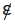## NCERT Solutions for Class 11 Maths Chapter 1 Sets Exercise 1.1

If you want Chapter 1 Sets Exercise 1.1 NCERT Solutions for Class 11 Maths then you're at right place. Here you will find Class 11 Maths NCERT Solutions that will help you building basic concepts about the topics that can be used to improve your marks in board exams. NCERT Solutions provided are prepared by Studyrankers experts that are accurate and detailed so you can use them to clear your doubts.

(i) The collection of all the months of a year beginning with the letter J.
(ii) The collection of ten most talented writers of India.
(iii) A team of eleven best-cricket batsmen of the world.
(iv) The collection of all boys in your class.
(v) The collection of all natural numbers less than 100.
(vi) A collection of novels written by the writer Munshi Prem Chand.
(vii) The collection of all even integers.
(viii) The collection of questions in this chapter.
(ix) A collection of most dangerous animals of the world.

(i) It is a set. The set of months in a year beginning with J is {January, June, July}.
(ii) The collection of ten most talented writers of India is not a set, because the term ‘most talented’ is not well defined.
(iii) A team of eleven best-cricket batsmen of the world is not a set, because the term ‘best-cricket batsman’ is not well defined.
(iv) The collection of all boys in your class is a set, because the boys in your class are welldefined.
(v) The collection of all natural numbers less than 100 is a set, because natural numbers less than 100 are {1, 2, 3,..., 99}.
(vi) The collection of novels written by the writer Munshi Prem Chand is a set, because this collection is well-defined.
(vii) The collection of all even integers is a set, because even integers are: {..., –4, –2, 0, 2, 4,..}.
(viii) The collection of questions in this chapter is a set, because the questions in this chapter are well-defined.
(ix) A collection of most dangerous animals of the world is not a set, because the term ‘most dangerous animal’ is not well defined.

2. Let A = {1, 2, 3, 4, 5, 6}. Insert the appropriate symbol ε or in the blank spaces:
(i) 5... A
(ii) 8... A
(iii) 0... A
(iv) 4... A
(v) 2... A
(vi) 10... A

(i) 5 εA
(iv) 4 εA
(v) 2 ε A
(vi) 10A

3. Write the following sets in roster form:
(i) A = {x: x is an integer and – 3 < x < 7}
(ii) B = {x: x is a natural number less than 6}
(iii) C = {x: x is a two-digit natural number such that the sum of its digits is 8}
(iv) D = {x: x is a prime number which is divisor of 60}
(v) E = The set of all letters in the word ‘TRIGONOMETRY’
(vi) F = The set of all letters in the word ‘BETTER’

(i) A = {–2, 1, 0, 1, 2, 3, 4, 5, 6}
(ii) B = {1, 2, 3, 4, 5}
(iii) C = {17, 26, 35, 44, 53, 62, 71, 80}
(iv) D = {2, 3, 5}
(v) E = {T, R, I, G, O, N, M, E, Y}
(vi) F = {B, E, T, R}.

4. Write the following sets in the set-builder form:
(i) {3, 6, 9, 12}
(ii) {2, 4, 8, 16, 32}
(iii) {5, 25, 125, 625}
(iv) {2, 4, 6,...}
(v) {1, 4, 9,... 100}

(i) A = {x: x = 3n, n ε N and 1 ≤ n ≤ 4}
(ii) B = {x: x = 2n, n ε N and 1 ≤ n ≤ 5}
(iii) C = {x: x = 5n, n ε N and 1≤ n ≤ 4}
(iv) D = {x: x is an even natural number}
(v) E = {x: x = n2, n ε N and 1 ≤ n ≤ 10}

5. List all the elements of the following sets:
(i) A = {x: x is an odd natural number}
(ii) B = {x: x is an integer, – 1/2 < x < 9/2}
(iii) C = {x: x is an integer, x2 ≤ 4}
(iv) D = {x: x is a letter in the word “LOYAL”}
(v) E = {x: x is a month of a year not having 31 days}
(vi) F = {x: x is a consonant in the English alphabet which precedes k}.

(i) A = {1, 3, 5,...}
(ii) B = {0, 1, 2, 3, 4}
(iii) C = {– 2, –1, 0, 1, 2}
(iv) D = {L, O, Y, A}
(v) E = {February, April, June, September, November}
(vi) F = {b, c, d, f, g, h, j}.

6. Match each of the set on the left in the roster form with the same set on the right described in set-builder form
 (i) {1, 2, 3, 6} (a) {x: x is a prime number and a divisor of 6} (ii) {2, 3} (b) {x: x is an odd natural number less than 10} (iii) {M, A, T, H, E, I, C, S} (c) {x: x is a natural number and divisor of 6} (iv) {1, 3, 5, 7, 9} (d) {x: x is a letter of the word